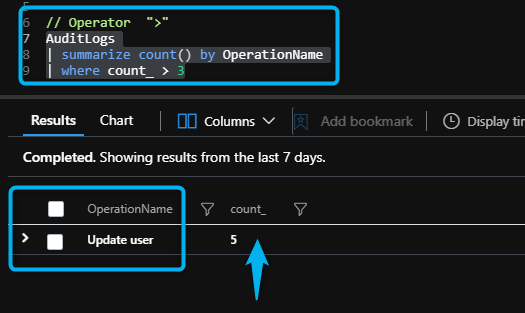# Learn KQL – Numerical Operators

In numerical analysis, a numerical method is a mathematical tool designed to solve numerical problems. The implementation of a numerical method with an appropriate convergence check-in in a programming language is called a numerical algorithm.

The types int, long, and real represent numerical types. The following operators can be used between pairs of these types:

Operator Description Example
`+` Add `3.14 + 3.14``ago(5m) + 5m`
`-` Subtract `0.23 - 0.22`,
`*` Multiply `1s * 5``2 * 2`
`/` Divide `10m / 1s``4 / 2`
`%` Modulo `4 % 2`
`<` Less `1 < 10``10sec < 1h``now() < datetime(2100-01-01)`
`>` Greater `0.23 > 0.22``10min > 1sec``now() > ago(1d)`
`==` Equals `1 == 1`
`!=` Not equals `1 != 0`
`<=` Less or Equal `4 <= 5`
`>=` Greater or Equal `5 >= 4`
`in` Equals to one of the elements
`!in` Not equals to any of the elements
Post Contents

## The Numerical Operators

Operator “<“

The “<” operator means “less”.

For example, if we run the following command and we’re interested in values that are less than 3, we need to run the following query:

AuditLogs
| summarize count() by OperationName
| where count_ < 3Another example is less 3 days by time generated with the following example:

OfficeActivity
| where TimeGenerated < ago(3d)

Operator “>”

The “>” operator means “greater”.

For example, if we run the command and we’re interested in values that are more than 3, we need to run the following query:

AuditLogs
| summarize count() by OperationName
| where count_ > 3Another example is less 3 days by time generated with the following example:

OfficeActivity
| where TimeGenerated > ago(3d)

Operator “<=”

The “<=” means “less or equal”.

For example, if we want values that are less or equals than 3, we need to run the following KQL query:

AuditLogs
| summarize count() by OperationName
| where count_ <= 3Operator “>=”

The “>=” operator means “greater or equals”.

For example, if we want values that are greater or equals than 3, we need to run the following KQL query:

AuditLogs
| summarize count() by OperationName
| where count_ >= 3# Learn KQL – Numerical Operators

In numerical analysis, a numerical method is a mathematical tool designed to solve numerical problems. The implementation of a numerical method with an appropriate convergence check-in in a programming language is called a numerical algorithm.
The types int, long, and real represent numerical types. The following operators can be used between pairs of these types:

Operator Description Example
`+` Add `3.14 + 3.14``ago(5m) + 5m`
`-` Subtract `0.23 - 0.22`,
`*` Multiply `1s * 5``2 * 2`
`/` Divide `10m / 1s``4 / 2`
`%` Modulo `4 % 2`
`<` Less `1 < 10``10sec < 1h``now() < datetime(2100-01-01)`
`>` Greater `0.23 > 0.22``10min > 1sec``now() > ago(1d)`
`==` Equals `1 == 1`
`!=` Not equals `1 != 0`
`<=` Less or Equal `4 <= 5`
`>=` Greater or Equal `5 >= 4`
`in` Equals to one of the elements
`!in` Not equals to any of the elements

## The Numerical Operators

Operator “<“
The “<” operator means “less”.
For example, if we run the following command and we’re interested in values that are less than 3, we need to run the following query:

AuditLogs
| summarize count() by OperationName
| where count_ < 3Another example is less 3 days by time generated with the following example:

OfficeActivity
| where TimeGenerated < ago(3d)

Operator “>”
The “>” operator means “greater”.
For example, if we run the command and we’re interested in values that are more than 3, we need to run the following query:

AuditLogs
| summarize count() by OperationName
| where count_ > 3Another example is less 3 days by time generated with the following example:

OfficeActivity
| where TimeGenerated > ago(3d)

Operator “<=”
The “<=” means “less or equal”.
For example, if we want values that are less or equals than 3, we need to run the following KQL query:

AuditLogs
| summarize count() by OperationName
| where count_ <= 3Operator “>=”
The “>=” operator means “greater or equals”.
For example, if we want values that are greater or equals than 3, we need to run the following KQL query:

AuditLogs
| summarize count() by OperationName
| where count_ >= 3